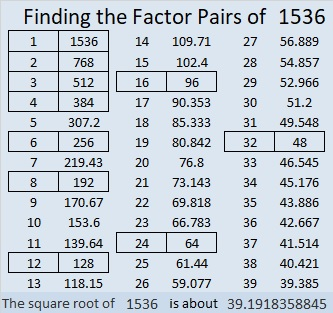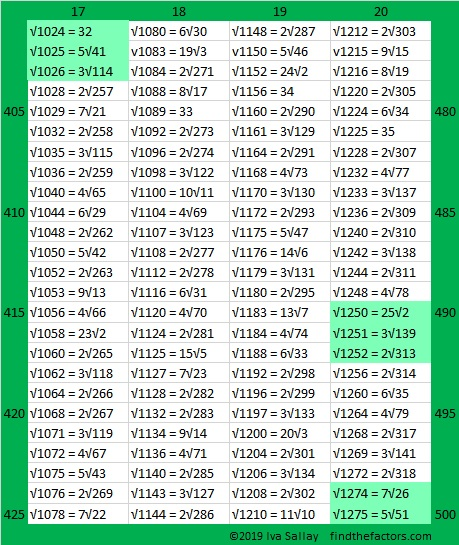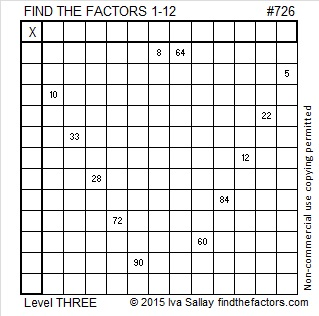# Factors of 1536 Make Sum-Difference!

### Today’s Puzzles:

1536 has 10 different factor pairs. One of those pairs adds up to 80 and a different one subtracts to give 80. Can you find the factor pairs that make sum-difference and write them in the puzzle? You can look at all of the factor pairs of 1536 in the graphic after the puzzle, but the second puzzle is really just the first puzzle in disguise. So try solving that easier puzzle first.That first puzzle is the first possible Sum-Difference puzzle. The second puzzle is only the 49th possible puzzle.

### Factors of 1536:

• 1536 is a composite number.
• Prime factorization: 1536 = 2 × 2 × 2 × 2 × 2 × 2 × 2 × 2 × 2 × 3, which can be written 1536 = 2⁹ × 3.
• 1536 has at least one exponent greater than 1 in its prime factorization so √1536 can be simplified. Taking the factor pair from the factor pair table below with the largest square number factor, we get √1536 = (√256)(√6) = 16√6
• The exponents in the prime factorization are 9 and 1. Adding one to each exponent and multiplying we get (9 + 1)(1 + 1) = 10 × 2 = 20. Therefore 1536 has exactly 20 factors.
• The factors of 1536 are outlined with their factor pair partners in the graphic below.### More About the Number 1536:

1536 is the difference of two squares in EIGHT different ways!
385² – 383² = 1536,
194² – 190² = 1536,
131² – 125² = 1536,
100² – 92² = 1536,
70² – 58² = 1536,
56² – 40² = 1536,
44² – 20² = 1536, and
40² – 8² = 1536.

1536 = 6 × 256.
256 is the 100th number whose square root can be simplified.
1536 is the 600th number whose square root can be simplified.

Here are the 501st to the 600th simplifiable square roots. If at least three square roots are consecutive, they are highlighted.# 1275 is the 50th Triangular Number AND the 500th Number Whose Square Root Can Be Simplified

I found two reasons to celebrate the number 1275: It is the 50th Triangular number, and it is the 500th number whose square root can be simplified.

First I’ll celebrate its square root by listing the 401st to the 500th numbers with simplifiable square roots. Having three or more simplifiable square roots in a row doesn’t happen that often, so I like to highlight them when it happens. 1274 and 1275 are highlighted because 1276 also has a square root that can be simplified:If you’re wondering what are the first 400 numbers with simplifiable square roots, you can click on the graphics below that will give you 100 at a time:

Now to celebrate that 1275 is the 50th triangular number, I’ve arranged \$12.75 in a triangle:1275 can also be evenly divided by 5, and 25, in other words, by nickels and quarters!

Nickels won’t make a triangle but they can form a trapezoid. Here’s how I made this one: 1275 ÷ 5 = 255 which is 300 (the 24th triangular number) minus 45 (the 9th triangular number). Thus we can make \$12.75 by arranging 255 nickels in a trapezoid with a top base of 10, a bottom base of 24, and a height of 15.We can also use quarters to make a trapezoid. Here’s what I did: 1275 ÷ 25 = 51 which is 66 (the 11th triangular number) minus 15 (the 5th triangular number). Thus, \$12.75 can be made by arranging 51 quarters in a trapezoid with a top base of 6, a bottom base of 11, and a height of 6.Can you find any rectangular ways to arrange the coins to total \$12.75?

Here’s a little more about the number 1275:

• 1275 is a composite number.
• Prime factorization: 1275 = 3 × 5 × 5 × 17, which can be written 1275 = 3 × 5² × 17
• The exponents in the prime factorization are 1, 2, and 1. Adding one to each and multiplying we get (1 + 1)(2 + 1)(1 + 1) = 2 × 3 × 2 = 12. Therefore 1275 has exactly 12 factors.
• Factors of 1275: 1, 3, 5, 15, 17, 25, 51, 75, 85, 255, 425, 1275
• Factor pairs: 1275 = 1 × 1275, 3 × 425, 5 × 255, 15 × 85, 17 × 75, or 25 × 51,
• Taking the factor pair with the largest square number factor, we get √1275 = (√25)(√51) = 5√51 ≈ 35.707141275 is the hypotenuse of SEVEN Pythagorean triples:
195-1260-1275 which is 15 times (13-84-85)
261-1248-1275 which is 3 times (87-416-425)
357-1224-1275 which is (7-24-25) times 51
540-1155-1275 which is 15 times (36-77-85)
600-1125-1275 which is (8-15-17) times 75
765-1020-1275 which is (3-4-5) times 255
891-912-1275 which is 3 times (297-304-425)

# Simplifying √726

• 726 is a composite number.
• Prime factorization: 726 = 2 x 3 x 11 x 11, which can be written 726 = 2 x 3 x (11^2)
• The exponents in the prime factorization are 1, 1, and 2. Adding one to each and multiplying we get (1 + 1)(1 + 1)(2 + 1) = 2 x 2 x 3 = 12. Therefore 726 has exactly 12 factors.
• Factors of 726: 1, 2, 3, 6, 11, 22, 33, 66, 121, 242, 363, 726
• Factor pairs: 726 = 1 x 726, 2 x 363, 3 x 242, 6 x 121, 11 x 66, or 22 x 33
• Taking the factor pair with the largest square number factor, we get √726 = (√121)(√6) = 11√6 ≈ 26.944387.Most numbers whose square roots can be simplified are divisible by 4, 9 or both. If I were trying to simplify √726, I would first apply divisibility tricks for 4 and 9 to the number 726:

• 726 is even so it is divisible by 2, but since 26 is not divisible by 4, neither is 726.
• Also notice that 7 + 2 + 6 = 15, a number divisible by 3, but not by 9. That means 726 is divisible by 3, but not by 9.

Thus 726 is NOT divisible by either 4 or 9. I will make a little cake to see if it is divisible by any other square numbers.

Dividing by 2 and then by 3 could be a good place to start, but rather than do those two divisions, I would simply do one division as I divide 726 by 6:I would immediately recognize that 121 is 11 × 11, so I don’t need to make any more layers for this factoring cake!

To simplify √726, take the square root of everything on the outside of this cake (the numbers in red): Thus, √726 = (√6)(√121) = 11√6.

——————————————————————

This level 3 puzzle is not difficult to do. If you start at the top of the first column and work down cell by cell, you’ll catch the rhythm of finding all the factors that can make this puzzle function as a multiplication table:Print the puzzles or type the solution on this excel file: 12 Factors 2015-12-28

—————————————————————

Here are a few more facts about the number 726:

726 is the average of 11^2 and 11^3 which is a characteristic that also makes it the 11th Pentagonal pyramidal number.

{724, 725, 726} is only the second set of three consecutive numbers in which each number in the set is the product of exactly one number squared and one different number. {603, 604, 605} was the first set.

726 is the sum of consecutive prime numbers 359 and 367.

726 is a palindrome in three bases:

• 10401 BASE 5; note that 1(625) + 0(125) + 4(25) + 0(5) + 1(1) = 726.
• 141 BASE 25; note that 1(625) + 4(25) + 1(1) = 726.
• MM BASE 32 (M = 22 base 10); note that 22(32) + 22(1) = 726.

——————————————————————